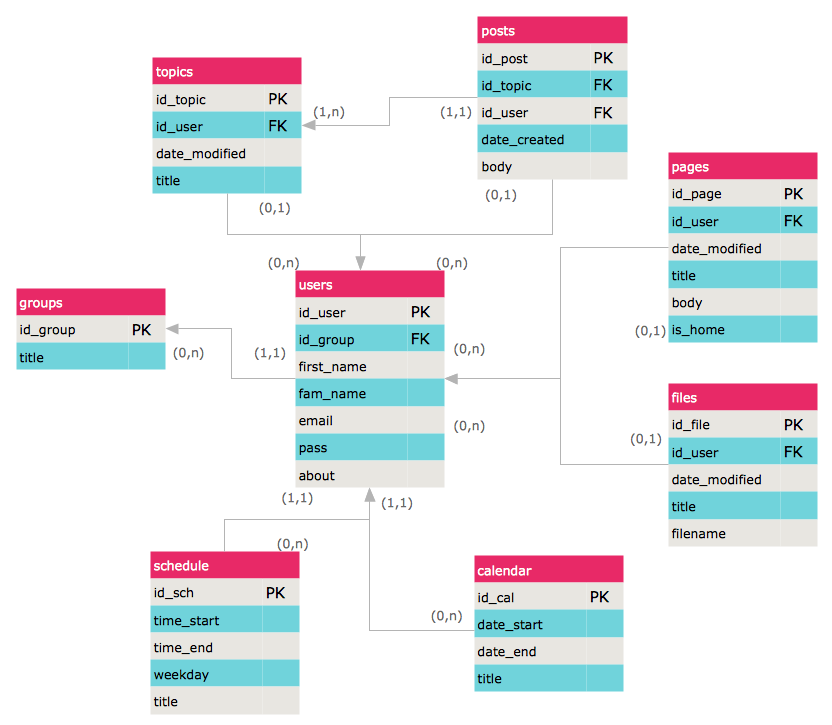This site uses cookies. By continuing to browse the ConceptDraw site you are agreeing to our Use of Site Cookies.
ConceptDraw Samples | Software development — ERD

# Entity-Relationship Diagram (ERD)

Samples of entity-relationship diagrams (ERD) are created using ConceptDraw DIAGRAM diagramming and vector drawing software enhanced with Entity-Relationship Diagram (ERD) solution from ConceptDraw Solution Park.

ConceptDraw DIAGRAM provides export of vector graphic multipage documents into multiple file formats: vector graphics (SVG, EMF, EPS), bitmap graphics (PNG, JPEG, GIF, BMP, TIFF), web documents (HTML, PDF), PowerPoint presentations (PPT), Adobe Flash (SWF).

## Tutorials and Solutions:

Video Tutorials: ConceptDraw Solution Park

Solutions: Entity-Relationship Diagram (ERD) for ConceptDraw DIAGRAM

## Sample 1: Entity-Relationship Diagram (ERD) — Chen's Notation

Entity-Relationship Diagram (ERD) sample: Chen's notation sample.

This example is created using ConceptDraw DIAGRAM diagramming and vector drawing software enhanced with Entity-Relationship Diagram (ERD) solution from ConceptDraw Solution Park.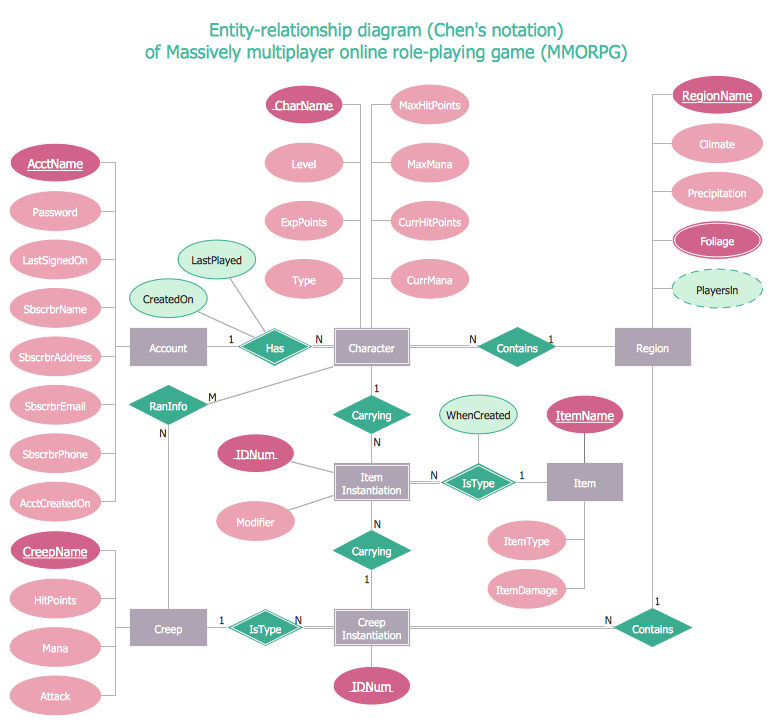## Sample 2: Entity-Relationship Diagram (ERD) — Crow's Foot Notation

Entity-Relationship Diagram (ERD): Crow's Foot notation sample.

This example is created using ConceptDraw DIAGRAM diagramming and vector drawing software enhanced with Entity-Relationship Diagram (ERD) solution from ConceptDraw Solution Park.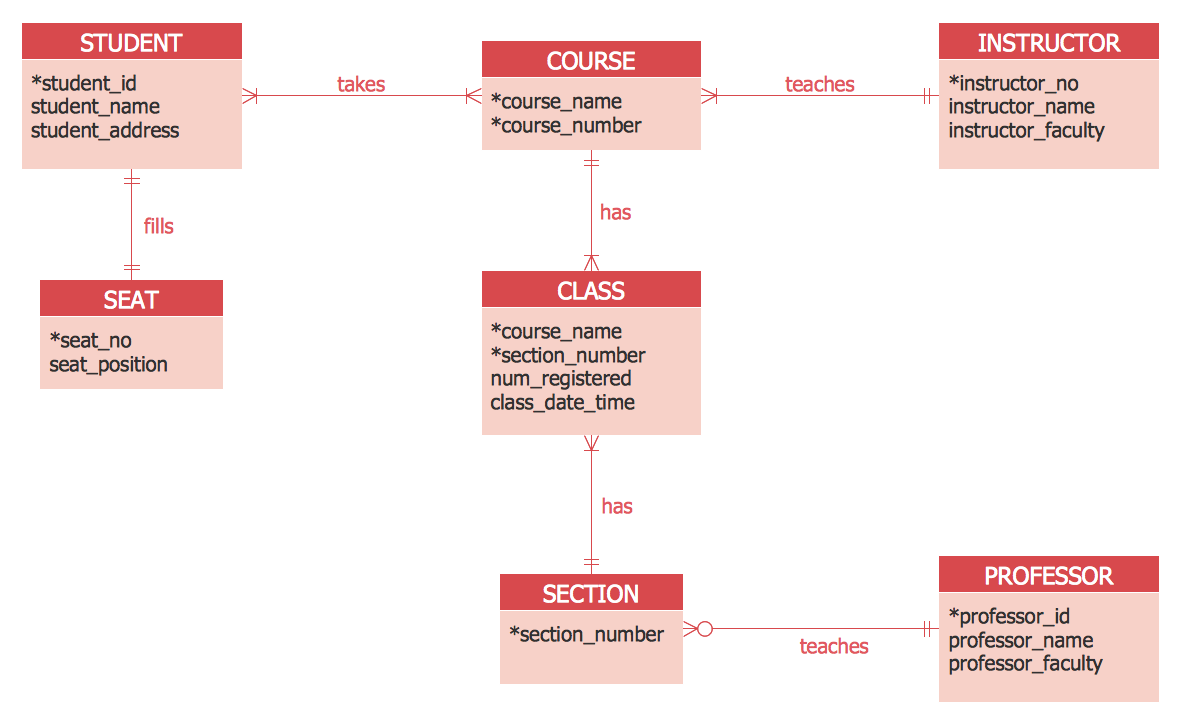## Sample 3: Entity-Relationship Diagram (ERD) — Students & Teachers

Entity-Relationship Diagram (ERD) sample - Students & teachers.

This example is created using ConceptDraw DIAGRAM diagramming and vector drawing software enhanced with Entity-Relationship Diagram (ERD) solution from ConceptDraw Solution Park.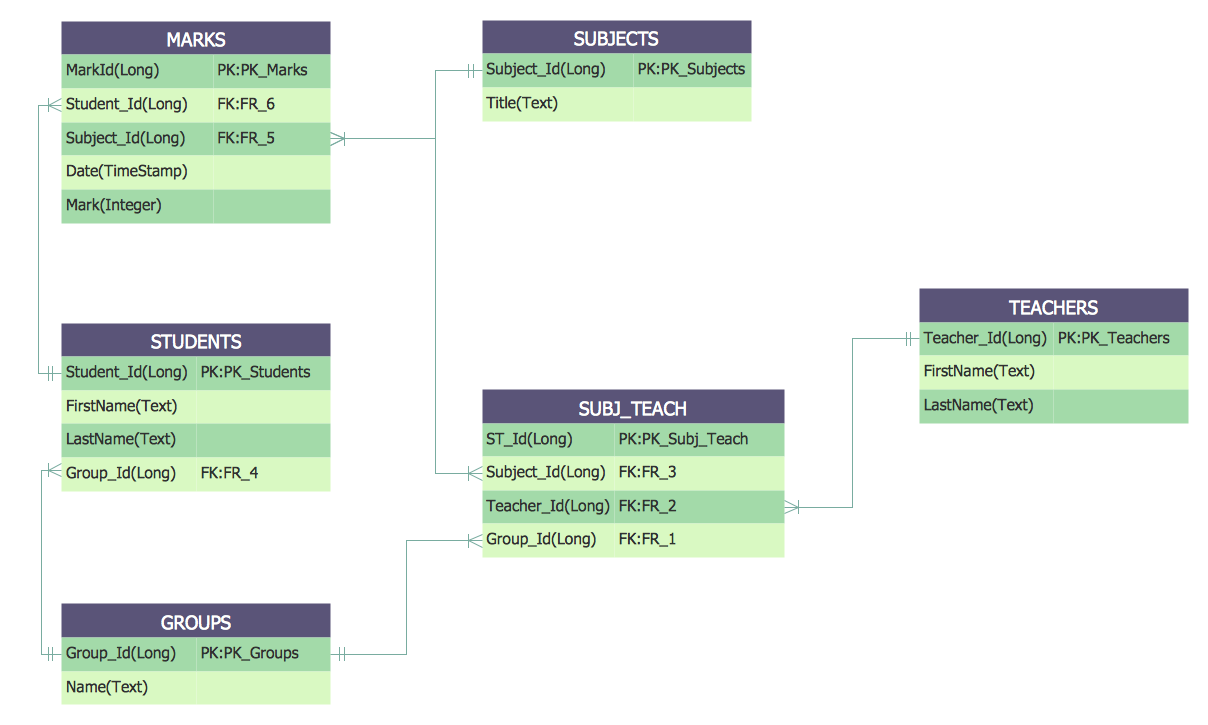## Sample 4: Entity-Relationship Diagram — WordPress File Reference

Entity-Relationship Diagram (ERD) sample: WordPress file reference.

This example is created using ConceptDraw DIAGRAM diagramming and vector drawing software enhanced with Entity-Relationship Diagram (ERD) solution from ConceptDraw Solution Park.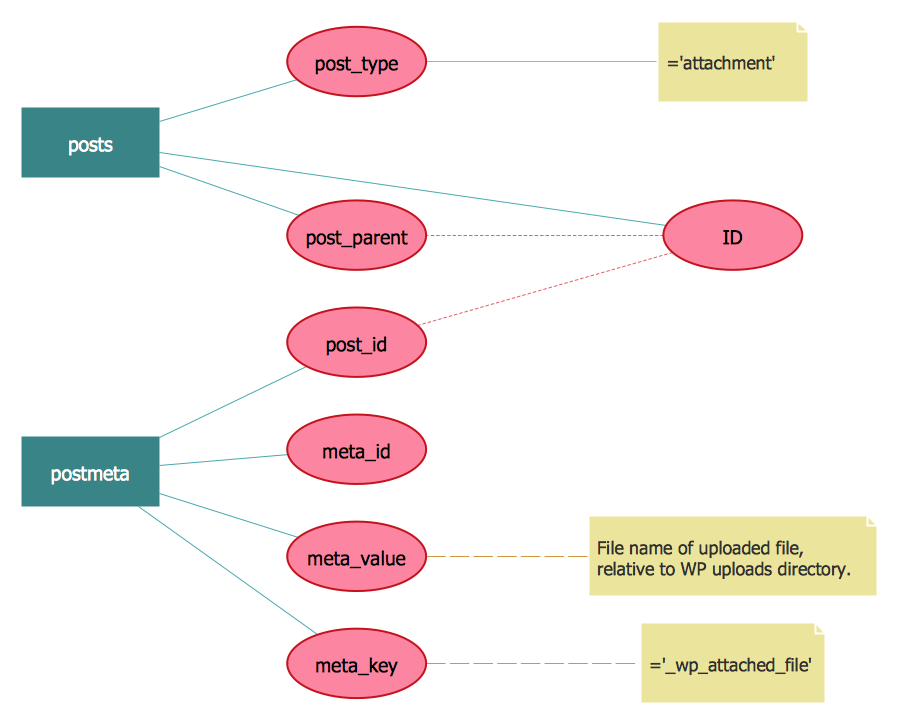## Sample 5: Entity-Relationship Model Diagram

Entity-Relationship Diagram (ERD) sample: Model diagram.

This example is created using ConceptDraw DIAGRAM diagramming and vector drawing software enhanced with Entity-Relationship Diagram (ERD) solution from ConceptDraw Solution Park.